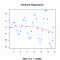I have forgotten

•http://facebook.com/
•https://www.google.com/accounts/o8/id
•https://me.yahoo.com

# Regression

#### Discrete

Approximates a discrete function using least squares polynomial fitting.
 double Discrete_once (int N, double *x, double *y, int degree, double a)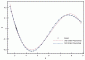#### Forsythe

Approximates an arbitrary function using Forsythe orthogonal polynomials.
 double Forsythe_once (int n, double *x, double *y, int degree, double a)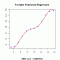#### Linear

Calculates the linear regression parameters and evaluates the regression line at arbitrary abscissas
 double Linear_once (int n, double *x, double *y, double a)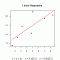#### Logistic

Evaluates the logistic regression curve built from a given set of points.
 double Logistic_once (int n, double *x, double *y, double a)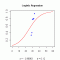#### Orthogonal

Approximates an arbitrary function using orthogonal polynomials.
 double Orthogonal_once (int n, double *x, double *y, int degree, double a)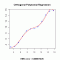#### Parabolic

Approximates an arbitrary function using parabolic least squares fitting.
 double Parabolic_once (int n, double *x, double *y, double a)#### Stiefel

Approximates a given function using the Stiefel-Remes method.
 double Stiefel_once (int n, double *x, double *y, int degree, double a)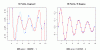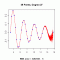#### Univariate Regression

univariate regression, polynomial regression, orthogonal polynomials, nonlinear